# Unfolding Naive Bayes From Scratch

Whether you are a beginner in Machine Learning or you have been trying hard to understand the Super Natural Machine Learning Algorithms and you still feel that the dots do not connect somehow, this post is definitely for you!

### Digging Deeper into the Mathematics of Probability

Now that you have built a basic understanding of the probabilistic calculations needed to train the Naive Bayes Model and then using it to predict probability for the given test sentence, I will now dig deeper into the probabilistic details.
While doing the calculations of probability of the given test sentence in the above section, we did nothing but implemented the given probabilistic formula for our prediction at test time: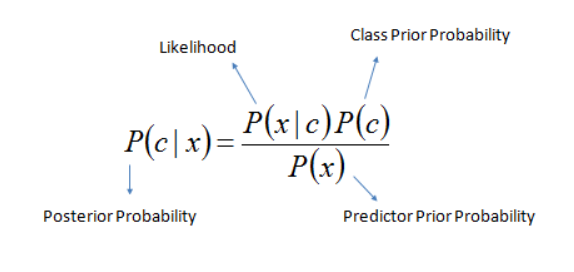Decoding the above mathematical equation :

“|” = refers to a state which has already been given / or some filtering criteria

“c” = class/category

“x” = test example/test sentence

A Quick Side Note : As like every other machine learning algorithm, Naive Bayes too needs a validation set to assess the trained model’s effectiveness. But , since this post was aimed to focus on the algorithmic insights, so I deliberately avoided it and directly jumped to the testing part

p (c|x) = given test example x, what is it’s probability of belonging to class c. This is also known as posterior probability. This is conditional probability that is to be found for the given test example x for each of the given training classes.

p(x|c) = given class c, what is the probability of example x belonging to class c. This is also known as likelihood as it implies how much likely does example x belongs to class c. This is conditional probability too as we are finding probability of x out of total instances of class c only i.e we have restricted/conditioned our search space to class c while finding the probability of x. We calculate this probability using the counts of words that are determined during the training phase.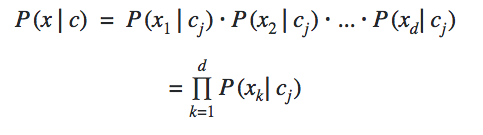Here “ j ” represents a class and k represents a feature
We implicitly used this formula twice above in the calculations sections as we had two classes. Remember finding the numerical value of product ( p of a test word “ j ” in class c
) ?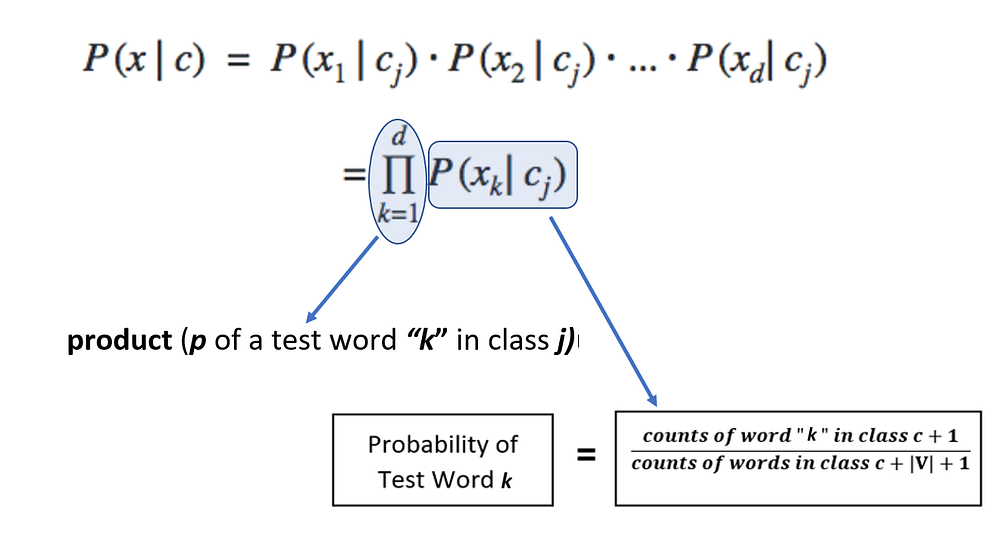p = This implies the probability of class c. This is also known as prior probability/unconditional probability. This is unconditional probability. We calculated this too earlier above in the probability calculations sections ( in Step # 1 which was finding value of term : p of class c )

p(x) = This is also known as normalizing constant so that the probability p(c|x) does actually falls in the range [0,1]. So if you remove this, the probability p(c|x) may not necessarily fall in the range of [0,1]. Intuitively this means probability of example x under any circumstances or irrespective of it’s class labels i.e whether positive or negative.
This is also reflected in total probability theorem which is used to calculate p(x) and dictates that to find p(x), we will find it’s probability in all given classes (because it is unconditional probability)and simply add them :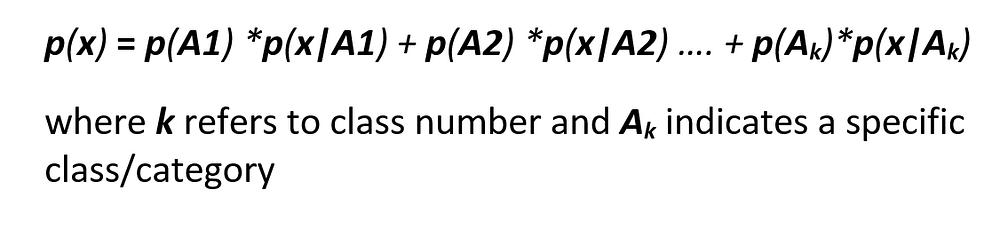Total Probability Theorem
This implies that if we have two classes then we would have two terms, so in our particular case of positive and negative sentiments: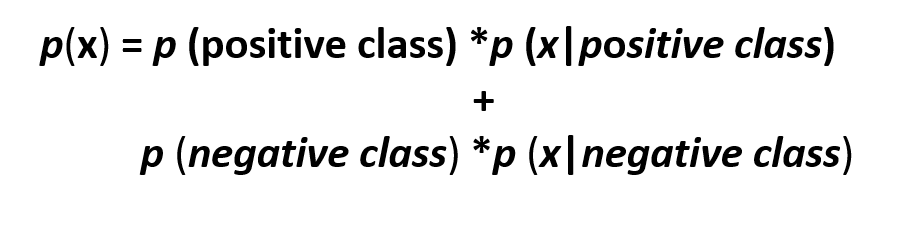Total Probability Theorem for Two Classes
Did we use it in the above calculations? No we did not. Why??? because we are comparing probabilities of positive and negative class and since the denominator remains the same, so in this particular case, omitting out the same denominator doesn’t affect the prediction by our trained model. It simply cancels out for both classes. So although we can include it but there is no such logical reason to do so. But again as we have eliminated the normalization constant, the probability p(c|x) may not necessarily fall in the range of [0,1]

Milestone # 4 Achieved 👍

### Avoiding the Common Pitfall of The Underflow Error!

• If you noticed, the numerical values of probabilities of words ( i.e p of a test word “ j ” in class c ) were quite small. And therefore, multiplying all these tiny probabilities to find product ( p of a test word “ j ” in class c ) will yield even a more smaller numerical value that often results in underflow which obviously means that for that given test sentence, the trained model will fail to predict it’s category/sentiment. So to avoid this underflow error, we take help of mathematical log as follows :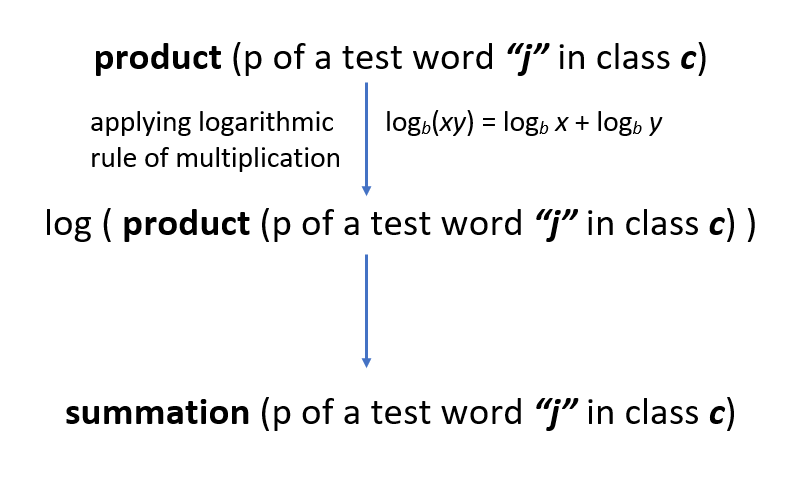Avoiding the Underflow Error
• So now instead of multiplication of the tiny individual word probabilities, we will simply add them. And why only log? why not any other function? Because log increases or decreases monotonically which means that it will not affect the order of probabilities. Probabilities that were smaller will still stay smaller after the log has been applied on them and vice versa. so let’s say that a test word “is” has a smaller probability than the test word “happy”, so after passing these through log would although increase their magnitude but “is” would still have a smaller probability than “happy”. Therefore, without affecting the predictions of our trained model, we can effectively avoid the common pitfall of underflow error.

Milestone # 5 Achieved 👍

### Concluding Notes….

• Although we live in a age of API’s and practically rarely code from scratch. But understanding the algorithmic theory in depth is extremely vital to develop a sound understanding of how the machine learning algorithms actually work. It is only the key understanding which actually differentiates a true data scientist from a naive one and what actually matters when training a really good model. So before moving to API’s , I personally believe that a true data scientist should code from scratch to actually see behind the numbers and the reason why a particular algorithm is better than the other.
• One of the best characteristics of the Naive Bayes Model is that you can improve it’s accuracy by simply updating it with new vocabulary words instead of always retraining it. You will just need to add words to the vocabulary and update the words counts accordingly. That’s it!Photo Credits : Benjamin Davies on Unsplash

At last! Finally! Milestone # 6 Achieved 😤 😤 😤

So that’s all for this blog post aaaand you have taken a step forward in you ML journey — cheers! 😄

Bio: Aisha Javed is a data scientist enthusiast interested in Deep Learning, Machine Learning, NLP and Kaggle.

Original. Reposted with permission.

Related:

By subscribing you accept KDnuggets Privacy Policy

By subscribing you accept KDnuggets Privacy Policy

By subscribing you accept KDnuggets Privacy Policy